Reach Us+44-1202068036
Statistical Methods and Software Package for Medical Trend Analysis in Health Rate Review Process | OMICS International
Journal of Health & Medical Informatics

Like us on:

All submissions of the EM system will be redirected to Online Manuscript Submission System. Authors are requested to submit articles directly to Online Manuscript Submission System of respective journal.

# Statistical Methods and Software Package for Medical Trend Analysis in Health Rate Review Process

Yin L1,2, Wu Q1 and Hong D1*

1Program of Actuarial Science, Middle Tennessee State University, Murfreesboro, TN, USA

2Department of Mathematics, University of Tennessee, Knoxiville, Tennessee, USA

*Corresponding Author:
Hong D
Program of Actuarial Science
Middle Tennessee State University
Murfreesboro, TN, USA
Tel: 615-904-8339
E-mail: [email protected]

Received date: December 21st 2015; Accepted date: March 5th 2016; Published date: March 15th 2016

Citation: Yin L, Wu Q, Hong D (2016) Statistical Methods and Software Package for Medical Trend Analysis in Health Rate Review Process. J Health Med Inform 7:219. doi: 10.4172/2157-7420.1000219

Copyright: © 2016 Yin L, et al. This is an open-access article distributed under the terms of the Creative Commons Attribution License, which permits unrestricted use, distribution, and reproduction in any medium, provided the original author and source are credited.

Visit for more related articles at Journal of Health & Medical Informatics

#### Abstract

Medical trend is the most important component used to indicate and file health insurance rates. Insurance companies apply trend analysis to forecast future costs and premiums. Governments use medical trend in the rate review process. In this paper we discuss four statistical methods: average ratio, linear regression, exponential regression and time series analysis, as well as their use in determining trend factors. An efficient method to detecting the outliers based on leave one out analysis is presented. A software package is developed within Microsoft Excel to calculate medical trend based on annual data or monthly data, which provides a useful tool for the insurance rate review.

#### Keywords

Medical trend analysis; Average ratio; Linear regression; Exponential regression; Autoregressive model; Outlier detection

#### Introduction

Medical costs keep growing and health spending continues to outpace the gross domestic product (GDP). The mean annual health insurance premium in the United States for employer-sponsored family coverage was \$13,770 in 2010. This equates to an increase of 114%, more than four times the rate of inflation over the past decade . The health insurance per family increased further to \$16,351 in 2013 , up 18% from year 2010. The increase of medical costs, or medical trends, is the single most important factor that causes health insurance rates to rise. Trend analysis uses historical experiences to project, or estimate, future experiences. In health rate review process, this analysis is vital in determining the reasonableness of proposed health insurance rates for a projected period.

Trend analysis methods

The primary purpose of medical trend analysis is to forecast future medical costs or claims. Insurance companies can use the forecasting to determine future health insurance premiums. Administrators can use the information to determine the reasonableness of the premiums charged by health insurers. Governments can use it to monitor the health systems. A variety of methods have been developed for trend analysis. These methods are not just limited to medical trend analysis, and can be used in many areas, such as climate change study  and quality control of healthcare improvements, cost of care trends, medical intervention assessment, etc.

Linear regression is a well-known statistical method that can be used for prediction and forecasting. The use of linear regression in trend analysis is summarized in . In linear regression, it is assumed that there is a linear relation between the detection and estimation. This relationship can be calculated either using the least square method or minimum absolute deviation method. The first method is more popular and has the advantage of easy implementation by solving a linear system, while the second one is more robust in cases that have outliers in the data set.

There are various extensions to linear regression method that can be used for trend analysis. Logistic regression and exponential regression are typical methods under the term of generalized linear models. These methods assume that the linear relationship exists between the transformed response and explanatory variables, instead of the original variables. It is more realistic to model the incremental pattern of the variable under investigation if the values of the variable are expected to grow exponentially (i.e., at a stable rate from period to period). This method has been used in analysis of climate and weather data  as well as maternal and child health insurance . Another regression model, Poisson regression, is also discussed in  when Rosenberg analyzed the trend in child health insurance. It assumes the response variable has a Poisson distribution. The advantage of Poisson regression in contrast to ordinary least squares regression is its ability of accounting for both the fluctuation across time and the variability at each time point.

In his trend analysis of large health administrative databases , Azimaee used inverse proportional function and negative exponential models to fit the data. These two methods are appropriate when the response variable is decreasing in time. Since we know medical costs keep growing, they are not suitable models for medical trend analysis. Analysis of Variance (ANOVA) is also a well-known statistical method that can be used for trend analysis when there are two or more samples. For example, the two-way ANOVA can be used when there are more than one independent variable and multiple observations for each independent variable. It has also been used in air pollution impact and trend analysis . This method however, does not emphasize on forecasting. Instead, it is useful to analyse the impact of the drivers to the overall trend.

Time series analysis is a very useful method for forecasting the future . A time series is a collection of observations of well-defined data items obtained through repeated measurements over time. Medical costs or claims are clear examples of time series data. Such data are usually correlated. Time series methods, like autoregressive models, can diagnose the precise nature of the correlation, adjust for it, and forecast the future values more accurately. Autoregressive models can be quite important in health care data where seasonal utilization and illness are a factor that can confound estimation of trend.

There are also non-parametric trend analysis methods. For example, Helsel and Hirsch used the Kendall-Theil method in their research of water resources. Aroner introduced Wilcoxon-Mann-Whitney Step Trend. Other advanced methods for trend interpretation to name a few, include the Triangular Episodic Presentation and Qualitative Scaling which is the generic methodology for qualitative analysis of the temporal shapes of process variables and non-parametric Mann- Kendall test and Sens Slope Estimator test to study the rainfall trend [9-13].

Background and motivation of this study

In 2011-2013, the Actuarial Science Program at Middle Tennessee State University (MTSU) was selected by the Tennessee Department of Commerce and Insurance (TDCI) for the rate review grant project based on the Patient Protection and Afford- able Care Act. During this project, the MTSU Actuarial Science Program examined several trend analysis methods that are potentially useful in rate review process.

Since so many methods for trend analysis have been proposed, the choice of the method is crucial for a specific application. Medical trend estimation is a complicated process. A typical method used in practice is to first estimate the changes of multiple components in medical costs (e.g. unit cost, utilization, mix of services, technology, etc.) and then apply them to calculate the medical trend. If the data volume is large enough, the actuary can further divide each component into subcomponents to achieve more accurate estimates. Clearly such kinds of extensive medical trend study require very detailed information from insurance carriers and are the task of the pricing actuary.

From a health review perspective, however, there are several reasons to focus on elementary statistical methods. First, as the insurance companies are reluctant to provide much details on their policies due to business confidentiality, this makes advanced analysis impossible. Second, the purpose of trend analysis for health rate review is to help administrators tell the reasonableness of premium increase request from health insurers, not to replicate their analyses. Finally, the Patient Protection and Affordable Care Act (PPACA) further protect the insured by the minimum medical loss ratio requirements. Therefore, a reference trend factor is usually sufficient to indicate the reasonableness of the premium increase request and would not hurt the interests of patients.

The health rate review has existed in Tennessee for a couple of years before the Affordable Care Act (ACA). The TDCI reviews rate changes from not only the small group market and individual market, but also large groups. The implementation of the ACA has changed the health insurance market. In particular, the individual market was dramatically changed dramatically - in particular, the individual market. This has imposed big challenges to the medical cost forecasting in the near future. More prospective insights on the impact of the ACA are required before the data become available. However, the medical trend estimate from historical data is still an important component. It is especially true for the small group market and large group market, which are less impacted by the ACA.

The purpose of this paper is to review several practical methods for the medical trend analysis, which are potentially useful in rate review process. The actual medical cost forecasting, which may be more involved and consider many adjustments in addition to the historical trend, is out of the scope of this paper.

Outline of this paper

Four trend analysis methods will be discussed in this paper: the average ratio method: linear regression, exponential regression, and time series analysis. In Section 2, we will discuss the requirements on the data that can be collected in the health review process and used in these methods. We review the use of the four trend analysis methods in Section 3. While all these methods are able to forecast the future medical costs, reporting the trend factor as a percentage cost increase in annual basis could be direct or indirect. A discussion is provided in Section 3.4. We developed a software package that codes all four methods and reports the trend factors. An introduction to this software package is given in Section 4. Medical cost data may be flawed for some reasons, and outliers can affect the accuracy of trend analysis. In Section 5, an outlier detection algorithm is proposed to help refine the data and improve the trend analysis. We close with summary and final remarks in Section 6.

#### Data

Before the forecasting process can begin, a reliable data set must be acquired to calculate an accurate result. Suitable data may be obtained from either the insurance companies or the government. Ideally, the historical and projected experiences should come from the same source. Moreover, the most appropriate data should be from the groups with the same policy or the groups with similar policies if aggregate trend analysis is performed.

The key consideration of time period is the length of the experience. The most recent 36 months to 48 months (3 years to 4 years) is typical. Shorter periods have several flaws. First, fewer data points are contained in a shorter time period, leading to unreliability and greater variability. Second, seasonal trends might appear as long term, as a short period cannot show the behavior of longer than the period examined seasonal effects.

Despite the law of large numbers, which states that increased data points yield more precise results, long term data also has its flaws. On one hand, finding data for 10 years or more is difficult; on the other hand, long term data cannot represent recent data very accurately with occurrence of severe downturns in economic, which causes more error in the forecasting result.

In elementary trend analysis for health review purposes, the data should at least contain earned premiums, incurred claims and memberships. Health insurance is a very complicated process. A thorough analysis needs details of the policy and consideration of many factors. However, on one hand, insurance companies are usually reluctant to provide too many details due to business confidential consideration. On the other hand, the purpose of medical trend analysis in the health rate review process is to provide a reference basis to determine the reasonableness of a filed premium increase request, not to replicate the analysis by the insurers. Therefore, the data is assumed to be sufficient if the claims and premium in as per member per month basis can be calculated for elementary trend analysis. The memberships are used in the analysis directly, but they are helpful in interpreting the reliableness of the analysis. The term memberships reflect how many members are under coverage by the company. Small membership coverage, for example an enrollment under

5000 will usually have more fluctuations, thereby increasing variability, causing more difficulties in analysis. It will need more advanced statistical methods in analysis to avoid reducing reliability.

Two types of data are most popular in medical insurance rate filing. The monthly data include the policy experience, which includes the number of enrollments, premiums and claims, for each month. The annual data includes summarized policy experience for each calendar year. When the data is annual, the trend analysis provides the annual trend factor. When the data is monthly, the trend analysis provides the monthly trend. To report the annualized trend, the following transformation could be used:

Annual Trend = (1 + Monthly Trend)12 − 1 (1)

Rolling average method for monthly data

For monthly data, seasonality is a very common phenomenon for healthcare claims. Neutralizing the fluctuation is quite important for the forecasting procedure. The preferred method to address the seasonality of medical claims is implementing calendar year data or a rolling 12 month method. When using the rolling 12 month method, each month’s value is the average of that month and the previous 11 month s’ values. Let Mibe the PMPM cost for the ith month. The rolling average value for the ith month is then calculated as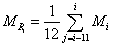As a rolling 12 month method was used to eliminate seasonality in the data, reversal is required for forecasted data points. More precisely, each monthly data point is the forecasted data point times 12 minus the sum of the 11 former month’s data. Let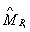denote the forecasted rolling average value for the ith month. Then

The foretasted monthly cost is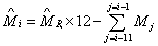In Figure 1, we plot the monthly data from a health carrier in Tennessee and the corresponding rolling average data. The solid line with square markers, which represents the cost per member per month, show obvious seasonal fluctuations. The medical cost at the end of each year is always much greater than the cost in the early months of the year. This is largely because of policy deductibles and partially because of seasonality of pandemics. The rolling average data, as represented by the dashed line, shows a clear increasing pattern. Since characterizing the seasonality of the data is not our purpose and the trend pattern is clearer in rolling average data, it is preferable to study rolling average data in trend analysis.

#### Methodologies

In this section, we will discuss the four methods for trend analysis: average ratio method, linear regression, exponential regression, and times series analysis.

Average ratio method

Medical costs have been seen to increase from time to time. In the average ratio method, we assume the expected medical costs increase by a constant factor from each period to its next period and the medical trend can be calculated as the rate of change in expected medical costs. In practice, however, real medical costs fluctuate and the rate of change could be different from time to time. The law of large numbers indicates that the average rate of change can be a good estimate for the expected rate of change, i.e., the trend factor.

Given a series of data points D1, D2. . . Dn, the rate of change from the ith time period to (i + 1) th time period is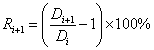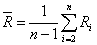Using this method to forecast the further claims is a little bit tricky. Theoretically, it could be done by the formulae E [Dn +1] = E [Dn] (1 + R¯). The problem here is that Dn may not be a good estimate of E [Dn] due to fluctuations. One possible fix is to use data of the last several (say, k) periods, project them to the last period, and estimate E[Dn] by the average, i.e.,Another solution is to use the information of the premiums and expected medical loss ratios of the carrier – their product usually reflects the best estimation of the claims.

In the average ratio method, the data is assumed to increase or decreases with a stable rate. If the medical cost has a sharp increase or decrease between two adjacent periods, or if the data has perpetual fluctuation during the observed time, then using the average ratio method will yield a large error used to estimate the 2014 year’s data according to the estimation formula, then the forecast will also contain the error in the year 2013 and errors accumulated before 2013. Therefore, the prediction may not represent a fully accurate result.

Regression methods

Regression is an approach to model the relationship between a dependent response variable and one or more explanatory variables. It considers data as a series, rather than consider each time point separately. The series view point is the most outstanding advantage of regression analysis. Regression is a common model to simulate the behavior of a data series; as it considers the errors, which are systematic and observable, generated by each data point. A regression model is therefore useful in predicting what is likely to happen in the next time period, or even in the far future. An additional advantage of the regression method is that it can account for multiple factors, which may affect the trend rate. For example, the multiple linear regression model 

Y = β 0 + β 1 X 1 + β 2X 2 + · · · + β k X k+ ε

Can contain k factors that may affect the medical trend, such as the average age of the insurance pool, the geographic factor, the average salary in a specific area, and so on.

To model the medical costs using regression method, we assume that the medical cost is a function of time. That is, the explanatory variable X is the date or the order number coding the date, and the response variable Y is the premium or medical claim cost. The simple linear regression model

Y = β 0 + β1 X + ε is applied, and we assume that the increase over each time period is a constant.

In reality, the exponential regression Y = exp (β 0 + β1 X + ε) is more preferable in medical trend analysis, as it assumes the increasing rate over the interval is a constant and medical cost always grows. An exponential regression is in a multiplicative manner, but it can be transformed to the simple linear model if we take the logarithmic value of both sides of the equation, which is ln (Y) = β 0 + β1 X + ε.

When the data is stable and the trend factor is not big (say < 15%), estimation by linear regression and exponential regression could be quite similar in the near future. To see this, we applied these two regression method to the data in Figure 1 and compare their forecasted values (see Figure 2.) It is seen that the prediction curves are very close to each other, even after 5 years. After a long time, there will be a clear difference between these two curves. However, the reliable forecast window will reach its limit before the difference emerges because longterm forecasts may contain more error. Therefore, linear and exponential regression does not have significant differences in shortterm forecasting, and either can satisfy the forecasting requirements of health rate review project.

Time series method

Unlike the simple average method and regression method, the time series method assumes the errors are correlated for some time period. Logically, the medical cost of a period must have some correlation with its former periods. One of the most obvious examples is that one person’s health situation is closely related to the last period - an unfortunate event may cause serious health problems for the next time period, and the effect may persist for a long time. One advantage of the time series method is that it can diagnose the precise nature of the correlation as well as adjust for it. The adjustments can narrow the range of the subsequent predicted values to produce a greater confidence band.

One typical model for time series analysis is the Autoregressive (AR) Model. The AR (p) model is a model that assumes the value Yt linearly depends on its p lagged values,

Yt = β 0 + β1 Yt − 1 + β2 Yt −2 + · · · + βp Yt − p + εt.

The appropriate p is determined by the Bayes information criterion (BIC) or Akaike Information criterion (AIC) , which minimizes the quantities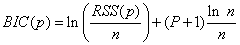or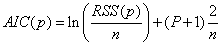respectively, where RSS(p) represents the sum of squares of residuals.

Although the purpose is to do trend analysis, the existence of trend could skew the the result of the AR models . The original data must be de trended first. We found that better results could be obtained if we use the transformed data in the AR model. Of course, for forecasting purpose, a reversal operation is needed to compute Dˆ n+1 from Yˆn +1.For the data we have collected during the Health Rate Review Project for the Tennessee Department of Commerce and Insurance, it seems the AR (1) model usually produces sufficiently stable results. Although this may not be the case for all other data sets, we do not bother considering more complicated models.

Yi= log (Di+1) – log (Di)

Report trend rate

Trend factor is usually reported as a percentage of increases in the claims or pre- miums in annual basis. For the average ratio method, the increasing rate is quite direct, it is just the average rate of increase. If the data is monthly, the monthly trend rate could be annualized using (1).

For the linear regression method, if the data is annual, the foretasted factor for the next year can be always calculated as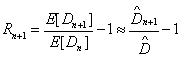Note that is the estimated value for time period n, that is, the expect value of Dn. As Dnmay inevitably contain fluctuation, using is more reliable in reporting the trend rate, since the residual error will be minimized during the regression procedure.

Note, however, the trend factor is different from period to period when the forecasting is made by linear regression. Thus, we cannot use (1) to annualized the trend. An alternative solution is to use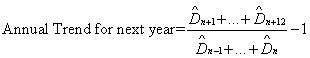For exponential regression, the trend rate can also be calculatedusing (2). How- ever, a simple alternative is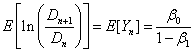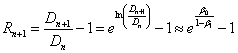It should be noted here that, unlike the linear trend regression model, the trend rate of exponential regression model is a constant, and it will not change with the time. As a result, annualized trend factor can be obtained by (1) from monthly trend factor.

For the time series analysis method, (2) is also useable. However, if the AR (1) model is applied to the transformed data Yi, a simple alternative exists.

#### Software Package

We developed a software package using the VBA language based on Microsoft Of- fice Excel. It coded all four methods: average ratio, linear regression, exponential regression and time series method to calculate the trend factor. Both data types, annual and monthly, are allowed. This software package is named the Medical Trend Calculator and can be run on both Windows systems and Mac systems. Figure 3 shows its interface, which includes a usage description and an access button.

By clicking the “ENTER” button, the trend calculator window willpop up, allowing the selection of data cell range and the data type. The trend factors calculated by the four methods are shown after clicking the “RUN” button. As an illustrative sample, Figure 4 shows the trend factors calculated for a health carrier in TN using annual data.

#### Outlier Detection

As mentioned before, for medical data, some experience periods are too long to provide currently meaningful trend information. Some data collection processes are not fully completed by the company when they file a premium increase request. The data sets may also be affected by an unexpected accident. The data will contain enough error to prevent an accurate calculation of the trend rate estimation. Therefore, to find a simple method that can detect these outliers efficiently is a critical componentto trend analysis. Note, however, the average ratio method and time series method do not allow removing outliers because they need consecutive data points.

A statistically reasonable method to detect outliers in the regression method is to compute the regression curve and study the residuals using a variety of methods proposed in . However, this method has an inevitable defect: the errors caused by outliers are inherent to the simulated behavior of the data. The outlier detection may be incorrect by using the regression curve that is flawed due to outliers.

To detect outliers in a data set, we propose a leave one out detection approach. To detect whether or not a point is an outlier, we run the regression without that data point, re-predict the value at that time point with the regression curve, compute the difference between the prediction and the original value, and represent it as a multiple of the stand error of that regression. When the original value is greater than the prediction, the multiple is a positive number; when the original value is less than the prediction, the multiple is a negative number. A point is regarded as an outlier with 95% confidence if the multiple lies outside of the interval −1.96 to 1.96. When there are several outliers, we only remove the most severe one to avoid a less severe one being mistakenly detected due to error brought in by other outliers. This data-cleaning process is repeated until there are no outlier left. The merit of such a method is that each regression can be performed without the outliers from the previous data set. The incremental reduction in outliers will increase the accuracy of the final regression and the resulting model.

We illustrate the use of the data cleaning process by an example shown in Table 1. The data set contains 11 data points, corresponding to the annual PMPM costs of 11 years. When the original data set is used, the four trend analysis methods output different trend factors, implying that the data is quite unreliable due to outliers. After the data cleaning process, the data becomes much more reliable and the four trend analysis methods give a similar trend factor.

Round 1 Round 2 Round 3 Round 4 Round 5
Multiple of Stand Error
Data 1 [red]−6.9911
Data 2 −0.1713 [red]−11.6806
Data 3 1.1408 0.5369 [red]−7.3918
Data 4 1.2670 1.3016 1.1837 −0.3375 0.2197
Data 5 0.9553 1.0356 1.2761 0.8491 1.3416
Data 6 0.5441 0.4980 0.1536 −1.1965 −1.4622
Data 7 0.2801 0.2646 0.0280 −0.8428 −1.2945
Data 8 0.1015 0.2162 0.5255 0.9384 0.6624
Data 9 −0.1546 0.0311 0.5996 2.0213 1.5983
Data 10 −0.5831 −0.4536 −0.2375 0.2596 −0.9156
Data 11 −1.1732 −1.1628 −1.6107 [red]−2.2725

Table 1: An example of the data-cleaning process.

From the table, we first recognize that the first data point is an outlier, then for the second round, we found that the second point is an outlier. Until round four, after removing the eleventh data point, all outliers are removed from the data set. Also, from the table, we can see that for each round, we do the regression without the error from the outlier that was detected in the former round. The merit of such a method is that the each regression can be performed without the outliers from the previous data set. The incremental reduction in outliers will increase the accuracy of the final regression and the resulting model.

#### Summary

In this paper, we have summarized four statistical methods for medical trend analysis: the average ratio method, the linear and exponential regression methods, and the autoregressive model. Although there are more advanced methods, these four methods are found to be simple but effective. In particular, they are sufficient to compute reference trend factors for use in the health rate review process. We also proposed an outlier detection method which iteratively removes outliers by the leave one out analysis.

To close, we would emphasize again that the pricing actuaries who have access to more detailed data do not simply use the trend rate on historical medical claims to forecast the future medical costs. Even for rate review purpose, we remark that the trend rate, as an annualized percentage increase in medical claims, is not the premium increasing rate, but a basis to calculate the premium increase. The actual premium increase depends on many other factors, for instance, the deductible leverage, the promotional business considerations, compliance with the law, the gap between historical experiences and projected period, and so on.

Note that each method provides unbiased and sufficient estimation when the assumptions for the corresponding model are satisfied. In practice, however, the fluctuations in the data are rarely pure noise and thus not completely random. This violates the model assumptions for all methods and makes the estimation biased. Things could be more complicated and worse when outliers (unusual fluctuations) occur. Our experiences tell that, if the model assumptions are not heavily violated and there are no significant outliers, all four methods provide similar results. In this case, every method can be sufficient for practical use. On the other side, if the results from these four methods differ a lot, we would suspect the data contains outliers. Manipulation is necessary until all four methods give similar result.

#### Acknowledgement

The authors would like to thank the anonymous referees for their valuable suggestions on the paper. Hong’s research was partially supported by Beijing Overseas Talents Program. We are also grateful to Brent Carpenetti at Blue Cross and Blue Shield of Tennessee and Brian Hoffmeister at the Tennessee Department of Comerce and Insurance for valuable discussions in the study.

#### References

Select your language of interest to view the total content in your interested language

### Article Usage

• Total views: 11462
• [From(publication date):
April-2016 - Aug 25, 2019]
• Breakdown by view type
• HTML page views : 11159
• PDF downloads : 303

## Post your commentCan't read the image? click here to refresh
###### Peer Reviewed Journals

Make the best use of Scientific Research and information from our 700 + peer reviewed, Open Access Journals

International Conferences 2019-20

Meet Inspiring Speakers and Experts at our 3000+ Global Annual Meetings

Top